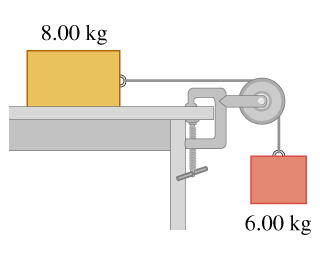# Problem: Consider the system shown in the figure . The rope and pulley have negligible mass, and the pulley is frictionless. Initially the 6.00-kg block is moving downward and the 8.00-kg block is moving to the right, both with a speed of 0.600 m/s . The blocks come to rest after moving 6.00 m .Use the work-energy theorem to calculate the coefficient of kinetic friction between the 8.00-kg block and the tabletop.

###### FREE Expert Solution
88% (293 ratings)
###### Problem Details

Consider the system shown in the figure. The rope and pulley have negligible mass, and the pulley is frictionless. Initially the 6.00-kg block is moving downward and the 8.00-kg block is moving to the right, both with a speed of 0.600 m/s . The blocks come to rest after moving 6.00 m .

Use the work-energy theorem to calculate the coefficient of kinetic friction between the 8.00-kg block and the tabletop.

Frequently Asked Questions

What scientific concept do you need to know in order to solve this problem?

Our tutors have indicated that to solve this problem you will need to apply the Energy in Connected Objects (Systems) concept. You can view video lessons to learn Energy in Connected Objects (Systems). Or if you need more Energy in Connected Objects (Systems) practice, you can also practice Energy in Connected Objects (Systems) practice problems.